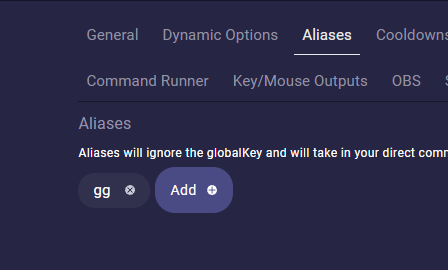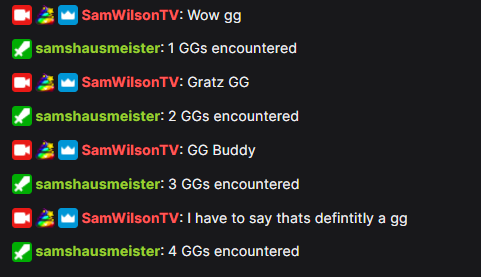# How to use the new Counter variable with Lumia Stream commands to create your death counter, GG counter and many more

Now you can count and Count von Count would be proud of youWe show you how to create a counter with Lumia Stream with an example of building a “GG counter”. Of course you can count everything what you want with it. Like deaths in a game, Use of Mana, how many energy drinks you had. There’s no limit to your creativity.

I.e. you want to count how often someone drops a “GG” (Good Game) in your chat you simply make your own variable to count these “GGs”. (Shoutout to MeldJ, who came up with the occasion)

No worries…it is not as hard as it sounds.

First, go to the Variables menu on the left sidebar. There you put the name you want to give that variable, a description of what it does and a start value. In this case, it is important to tick the Counter checkbox.

Then we create a command. Go to Commands->Chat and click “Add Command”.
I call this command simply “count” with the type “No light change” and I untick “Show this command in the !commands page”

As we want the command to react on “GG” and not on “!count” we will give this command an Alias. Go to the tab alias and put gg in it.
` This is optional and depends on your needs.`This was just the basic structure, the magic comes now with the tab “Lumia Actions”. Head to Lumia Actions and set `Type` to Update Variable, in `Variable` you choose your fresh own variable from the dropdown, in my case the counter variable, and in `Value`, we put … nothing because a blank field will increment by 1.

You can choose any of the basic arithmetic operations.
leave blank: will increment by 1,
number: will increment by the number, so if the number was 10 and value is 20 it will equal 30
“+” number: will increment by the number, so if the number was 10 and value is + 20 it will equal 30
“-” number: will subtract by the number, so if the number was 10 and value is - 4 it will equal 6
“/” number: will divide by the number, so if the number was 10 and value is / 2 it will equal 5
“*” number: will multiply by the number, so if the number was 10 and value is * 2 it will equal 20
“%” number: will modulus by the number, so if the number was 10 and value is % 9 it will equal 1
“=” number: will set equal to the number, so if the number was 10 and value is = 2 it will equal 2

Last but not least we prepare our ChatBot to react on a GG. For this, we go to the ChatBot tab and put a nice chat message in it. To see our count we use the built variable {{counter}}

And that’s it. Now your bot will count every nicely or not so nicely meant GG which comes to your Chat